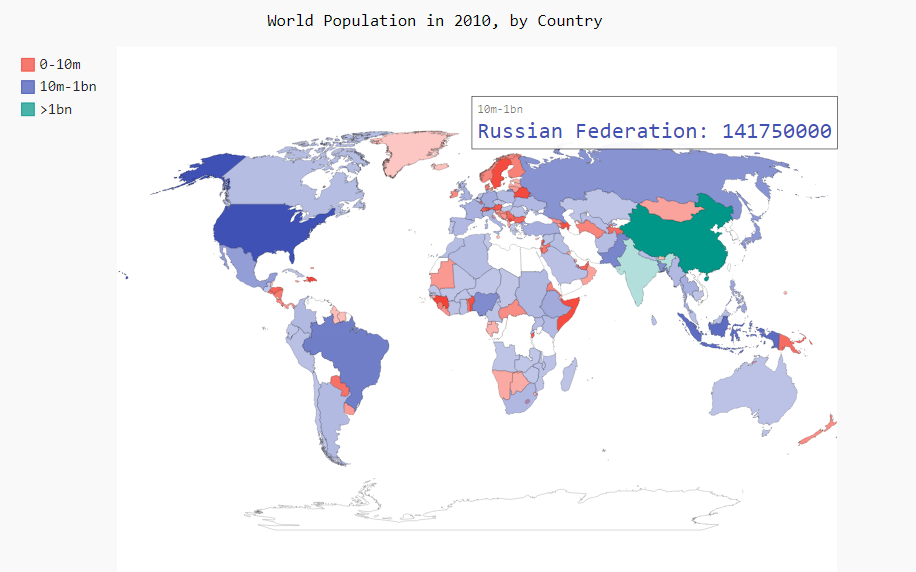### 16 使用Python下载数据

16.1 csv文件格式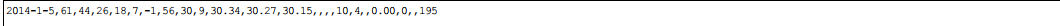# 16.1.1 分析CSV文件头

``filename = 'file/sitka_weather_07-2014.csv'with open(filename) as f:    reader = csv.reader(f)    hreader_row = next(reader)    print(hreader_row)``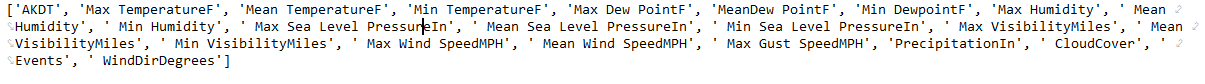# 16.1.2 打印文件头及其位置
# 为了让文件头数据更容易让人理解,将列表中每个文件头以及位置打印出来

``filename = 'file/sitka_weather_07-2014.csv'with open(filename) as f:    reader = csv.reader(f)    hreader_row = next(reader)     for index, colum_hreader in enumerate(hreader_row):        print(index, colum_hreader)``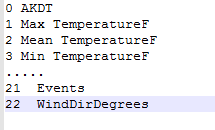# 16.1.3 提取并读取数据

``# 知道哪些数据后,读取每天的的最高气温# 从文件中读取最高气温filename = 'file/sitka_weather_07-2014.csv'with open(filename) as f:    reader = csv.reader(f)    hreader_row = next(reader)    # 创建一个highs的空列表,在遍历文件余下的各行    highs = []    # 遍历文件中余下的各行    for row in reader:        # 将字符串转为数字        highs.append(row)    print(highs)``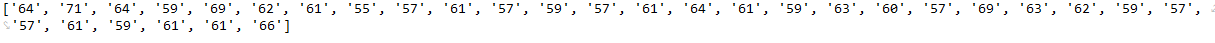``... for row in reader:        # 将字符串转为数字	high = int(row)         highs.append(high)...``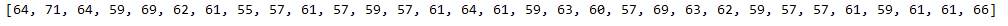# 16.1.4 绘制气温图表

``#从文件中获取最高气温...# 根据数据绘制图形# figsize:指定figure的宽和高，单位为英寸；fig = plt.figure(dpi=128, figsize=(10, 6))plt.plot(highs, c='red')# 设置图形的格式plt.title("Dailly high temperatures July 2014")# 设置名称plt.xlabel('x', fontsize=16)plt.ylabel('Temperature(F)', fontsize=16)plt.tick_params(axis='both', which='major', labelsize=16)plt.show()``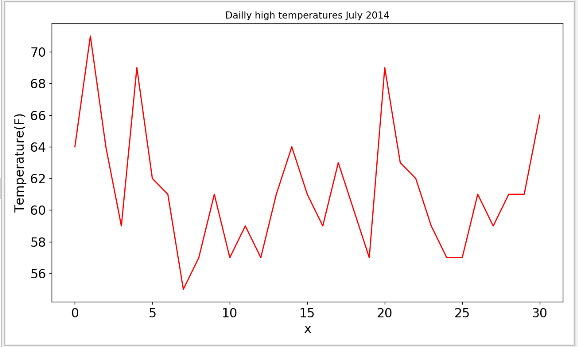# 16.1.5模块datetime

``注意:导入datetime时,不能省略from datetimefrom datetime import datetime# 我们首先导入了模块datetime中的datetime类，然后调用方法strptime()，并将包含所需日期的字符串作为第一个实参。# 第二个实参告诉Python如何设置日期的格式。在这个示例中，' %Y-，让Python将字符串中第一个连字符前面的部分视为四位的年份:#  %m，让Python将 第s个连字符前面的部分视为表示月份的数字:而’%d’让Python将字符串的最后-部分视为月份中的一天(1~31) 。# 方法strptime()可接受各种实参，并根据它们来决定如何解读日期。 first_date = datetime.strptime('2014-7-1', '%Y-%m-%d')``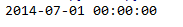# 16.1.6在图表中添加日期

``filename = 'file/sitka_weather_07-2014.csv'with open(filename) as f:    reader = csv.reader(f)    hreader_row = next(reader)    # 创建一个highs的空列表,在遍历文件余下的各行    highs = []    # 从文件中提取日期,    dates = []    # 遍历文件中余下的各行    for row in reader:        # 将字符串转为数字        high = int(row)        highs.append(high)        current_date = datetime.strptime(row, '%Y-%m-%d')        dates.append(current_date)    print(highs) # 根据数据绘制图形# figsize:指定figure的宽和高，单位为英寸；fig = plt.figure(dpi=128, figsize=(10, 6))# 将日期和最高气温值传递给plotplt.plot(dates, highs, c='red')# 设置图形的格式plt.title("Dailly high temperatures July 2014")# 设置名称plt.xlabel('x', fontsize=16)plt.ylabel('Temperature(F)', fontsize=16)# 绘制斜的日期标签,以免他们重叠fig.autofmt_xdate()plt.tick_params(axis='both', which='major', labelsize=16)plt.show()``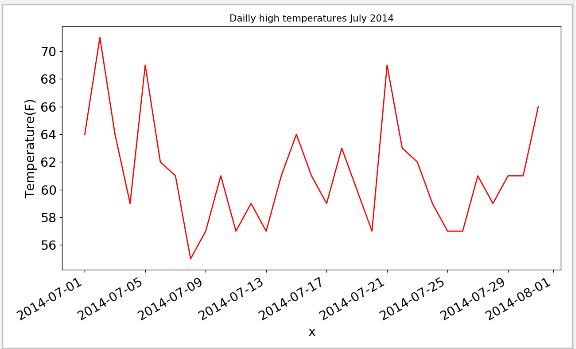# 16.1.7 涵盖更长的时间

``filename = 'file/sitka_weather_2014.csv'with open(filename) as f:	...# 设置图形的格式plt.title("Dailly high temperatures - 2014")# 设置名称plt.xlabel('x', fontsize=16)``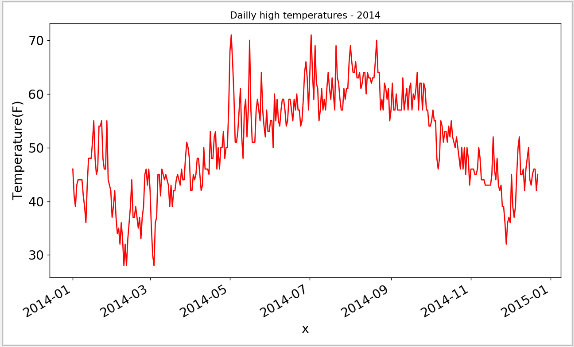# 16.1.8 再绘制一个数据系列

# 上图显示了大量意义深远的数据，但我们可以在其中再添加最低气温数据，使其更有用。
# 为此，需要从数据文件中提取最低气温，并将它们添加到图表

``import csvfrom datetime import datetimefrom matplotlib import pyplot as plt filename = 'file/sitka_weather_2014.csv'with open(filename) as f:    reader = csv.reader(f)    header_row = next(reader)    dates, highs, lows = [], [], []    for row in reader:        current_date = datetime.strptime(row, '%Y-%m-%d')        dates.append(current_date)        high = int(row)        low = int(row)        highs.append(high)        # 储存最低气温        lows.append(low)# 根据数据绘制图形fig = plt.figure(dpi=128, figsize=(10, 6))plt.plot(dates, highs, c='red')# 绘制最低气温plt.plot(dates, lows, c='blue')# 设置图形的格式plt.title("Dailly high and low temperatures - 2014")# 设置名称plt.xlabel('x', fontsize=16)plt.ylabel('Temperature(F)', fontsize=16)# 绘制斜的日期标签,以免他们重叠fig.autofmt_xdate()plt.tick_params(axis='both', which='major', labelsize=16)plt.show()``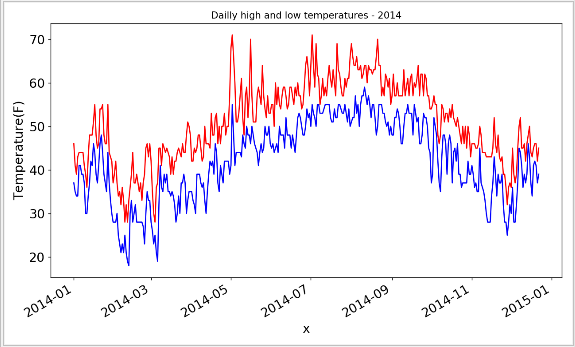# 16.1.9 给图表区域着色
# 添加两个数据系列后，我们就可以了解每天的气温范围了。下面来给这个图表做最后的修饰，通过着色来呈现每天的气温范围。
# 为此，我们将使用方法fill_between() ，它接受一个 x 值系列和两个 y 值系列，并填充两个 y 值系列之间的空间

``读取文件值...# 根据数据绘制图形fig = plt.figure(dpi=128, figsize=(10, 6))# alpha 指定颜色透明度,Alpha值为0表示完全透明,1默认完全不透明plt.plot(dates, highs, c='red', alpha=0.5)# 绘制最低气温plt.plot(dates, lows, c='blue', alpha=0.5)# 我们向fill_between() 传递了一个 x 值系列：列表dates ，还传递了两个 y 值系列：highs 和lows 。# 实参facecolor 指定了填充区域的颜色，我们还将alpha 设置成了较小的值0.1，# 让填充区域将两个数据系列连接起来;plt.fill_between(dates, highs, lows, facecolor='blue', alpha=0.1)``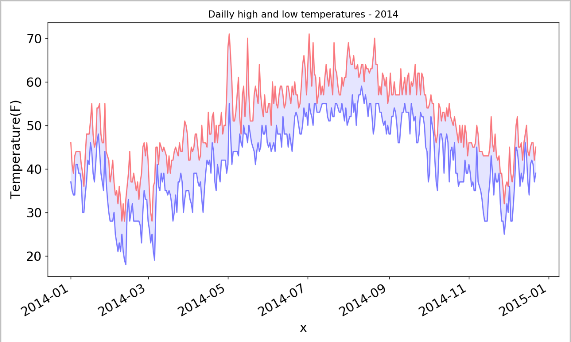# 16.1.10 错误检查

# 我们应该能够使用有关任何地方的天气数据来运行highs_lows.py中的代码，
# 但有些气象站会偶尔出现故障，未能收集部分或全部其应该收集的数据。缺失数据可能会引发异常，
# 如果不妥善地处理，还可能导致程序崩溃。
# 例如，我们来看看生成加利福尼亚死亡谷的气温图时出现的情况。将文件death_valley_2014.csv(下载)复制到工程目录

``filename = 'file/death_valley_2014.csv'with open(filename)  as f:    reader = csv.reader(f)    header_row = next(reader)    dates, highs, lows = [], [], []    for row in reader:        current_date = datetime.strptime(row, '%Y-%m-%d')        dates.append(current_date)        high = int(row)        low = int(row)        highs.append(high)        # 储存最低气温        lows.append(low)``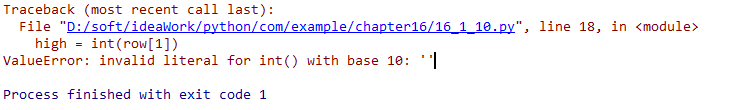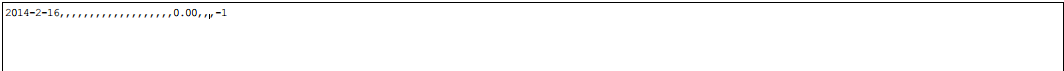``filename = 'file/death_valley_2014.csv'with open(filename)  as f:    reader = csv.reader(f)    header_row = next(reader)    dates, highs, lows = [], [], []    for row in reader:        try:            current_date = datetime.strptime(row, '%Y-%m-%d')            high = int(row)            low = int(row)        except ValueError:            print(current_date, 'missing data')        else:            dates.append(current_date)            highs.append(high)            # 储存最低气温            lows.append(low)...# 根据数据绘制图形..``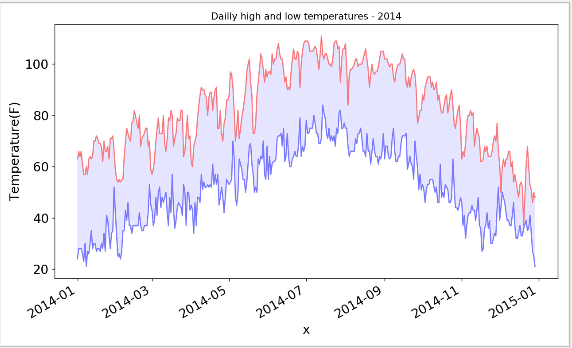16.2 制制作作世世界界人人口口地地图图：JSON格式

16.2.1 下载世界人口数据(population_data下载)

16.2.2 提取2010年每个国家的人口数量

``filename = 'file/population_data.json'with open(filename) as f:    pop_data = json.load(f) # 打印每个国家2010年的人口数量for pop_dict in pop_data:    if pop_dict['Year'] == '2010':        country_name = pop_dict['Country Name']        population = pop_dict['Value']        print("country_name:" + country_name + ":" + "population:" + population)``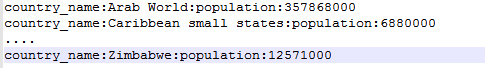# 16.2.3 将字符串转为数字值

``filename = 'file/population_data.json'with open(filename) as f:    pop_data = json.load(f)# 打印每个国家2010年的人口数量for pop_dict in pop_data:    if pop_dict['Year'] == '2010':        country_name = pop_dict['Country Name']        # Python不能将字符串'1127437398.85751' 转换为整数,为了消除这种错误,        # 我们先将字符串转换为浮点数,在将浮点数转换为整数        population = int(float(pop_dict['Value']))        print("country_name:" + str(country_name) + ":" + "population:" +              str(population))``

# 16.2.4 获取两个字母的国别码

# 制作地图前，还需要解决数据存在的最后一个问题。Pygal中的地图制作工具要求数据为特定的格式：
# 用国别码表示国家，以及用数字表示人口数量。处理地理政治数据时，经常
# 需要用到几个标准化国别码集。population_data.json中包含的是三个字母的国别码，但Pygal
# 使用两个字母的国别码。我们需要想办法根据国家名获取两个字母的国别码
# Python3使用时需要在终端使用pip3指令进行安装
# pip install pygal_maps_world

``for country_code in sorted(COUNTRIES.keys()):    print(country_code, COUNTRIES[country_code])``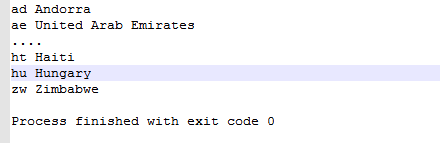# 为获取国别码，我们将编写一个函数，它在COUNTRIES 中查找并返回国别码。
# 我们将这个函数放在一个名为country_codes 的模块中，以便能够在可视化程序中导入它

``# 为获取国别码，我们将编写一个函数，它在COUNTRIES 中查找并返回国别码。# 我们将这个函数放在一个名为country_codes 的模块中，以便能够在可视化程序中导入它def get_country_code(country_name):    """根据指定的国家,返回Pygal使用的两个字母的国别码"""    for code, name in COUNTRIES.items():        if name == country_name:            return code     # 如果没有找到指定的国家,就返回NONE    return None 提取国家名和人口数量filename = 'file/population_data.json'with open(filename) as f:    pop_data = json.load(f) for pop_dict in pop_data:    if pop_dict['Year'] == '2010':        country_name = pop_dict['Country Name']        population = int(float(pop_dict['Value']))        code = get_country_code(country_name)        if code:            print(code + ":" + str(population))        else:            print("Error-" + country_name)``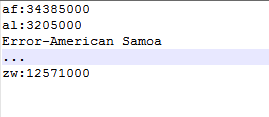16.2.5 制作世界地图

有了国别码后，制作世界地图易如反掌。Pygal提供了图表类型Worldmap ，可帮助你制作呈现各国数据的世界地图。
# 为演示如何使用Worldmap ，我们来创建一个突出北美、中美和南美的简单地图

``import pygal_maps_world.maps wm = pygal_maps_world.maps.World()wm._title = 'North,Central, and South America'wm.add('North America', ['ca', 'mx', 'us'])wm.add('Central America', ['bz', 'cr', 'gt', 'hn', 'ni', 'pa', 'sv'])wm.add('South America', ['ar', 'bo', 'br', 'cl', 'co', 'ec', 'gf', 'gy',                         'pe', 'py', 'sz', 'uy', 've'])wm.render_to_file('americas.svg')``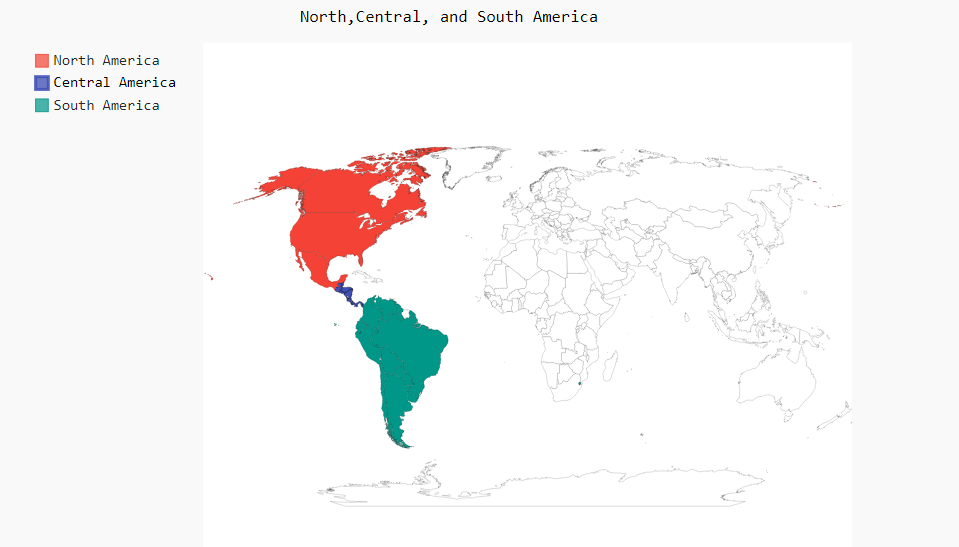# 16.2.6 在世界地图上呈字

``wm = pygal_maps_world.maps.World()wm.title = 'North,Central, and South America'wm.add('North America', {'ca': 34126000, 'us': 309349000, 'mx': 113423000})# 首先，创建了一个Worldmap 实例并设置了标题。接下来，使用了方法add() ，# 但这次通过第二个实参传递了一个字典而不是列表。这个字典将两个字母的Pygal国别码作为键# 将人口数量作为值。Pygal根据这些数字自动给不同国家着以深浅不一的颜色（人口最少的国家颜色最浅，人口最多的国家颜色最深），wm.render_to_file('americas.svg')``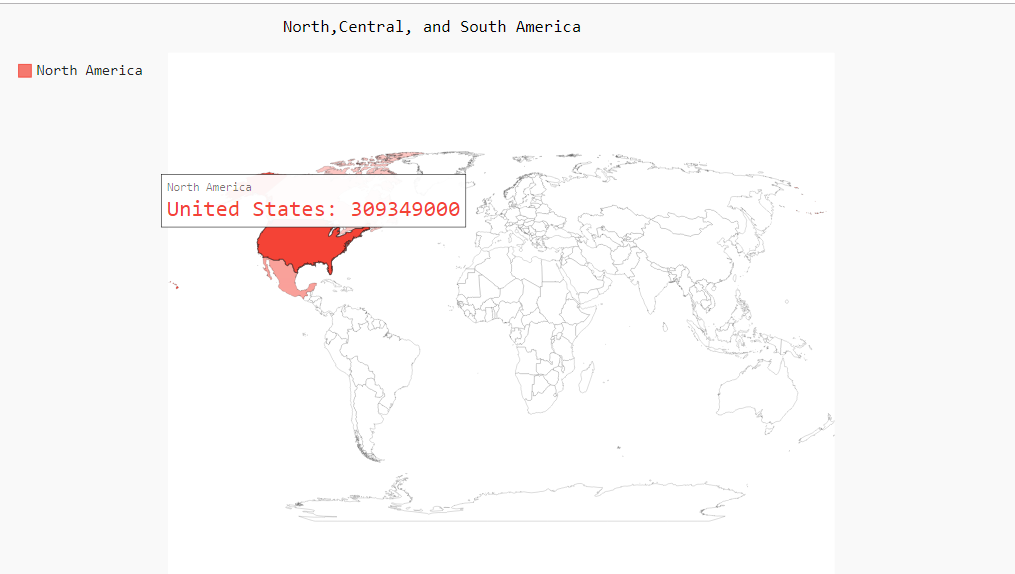# 16.2.7 绘制完整的世界人口地图

``将数据加载到列表中...cc_populations = {}for pop_dict in pop_data:    if pop_dict['Year'] == '2010':        country_name = pop_dict['Country Name']        population = int(float(pop_dict['Value']))        code = get_country_code(country_name)        if code:            cc_populations[code] = population wm = pygal_maps_world.maps.World()wm.title = 'World population in 2010,by Country'wm.add('2010', cc_populations)wm.render_to_file("world_population.svg")``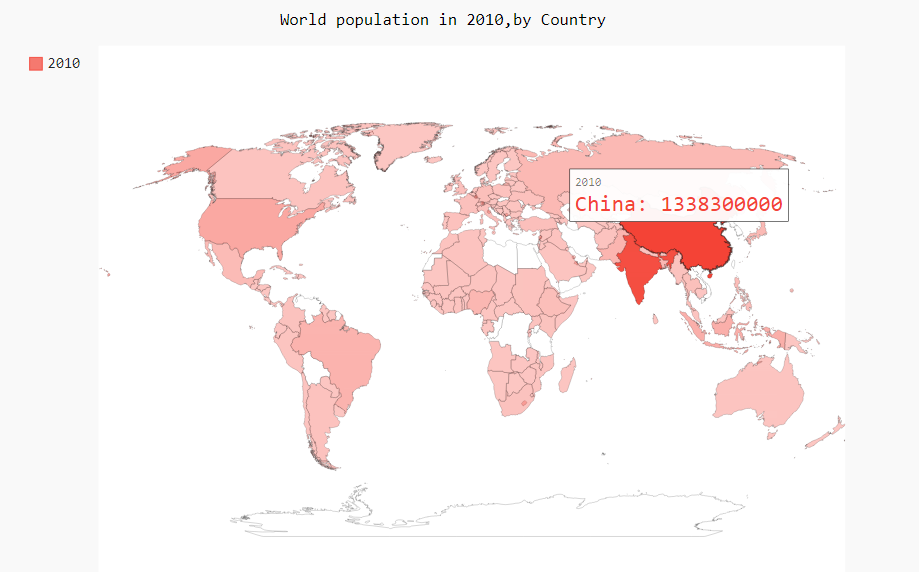# 16.2.8 根据人口数量将国家分组

# 印度和中国的人口比其他国家多得多，但在当前的地图中，它们的颜色与其他国家差别较小。
# 中国和印度的人口都超过了10亿，接下来人口最多的国家是美国，但只有大约3亿。
# 下面不将所有国家都作为一个编组，而是根据人口数量分成三组——少于1000万的、介于1000万和10亿之间的以及超过10亿的：

``创建一个人口的字典# 根据人口数量将所有的国家分成三组cc_pops_1, cc_pops_2, cc_pops_3 = {}, {}, {}for cc, pop in cc_populations.items():    if pop < 10000000:        cc_pops_1[cc] = pop    if pop < 1000000000:        cc_pops_2[cc] = pop    else:        cc_pops_3[cc] = pop # 看看每组包含多少个国家print(    "每组包含多少个国家:" + str(len(cc_pops_1)) + " " + str(len(cc_pops_2)) + " " + str(        len(cc_pops_3)))wm = pygal_maps_world.maps.World()wm.title = 'World Population in 2010, by Country'wm.add('0-10m', cc_pops_1)wm.add('10m-1bn', cc_pops_2)wm.add('>1bn', cc_pops_3)wm.render_to_file('world_population.svg')``